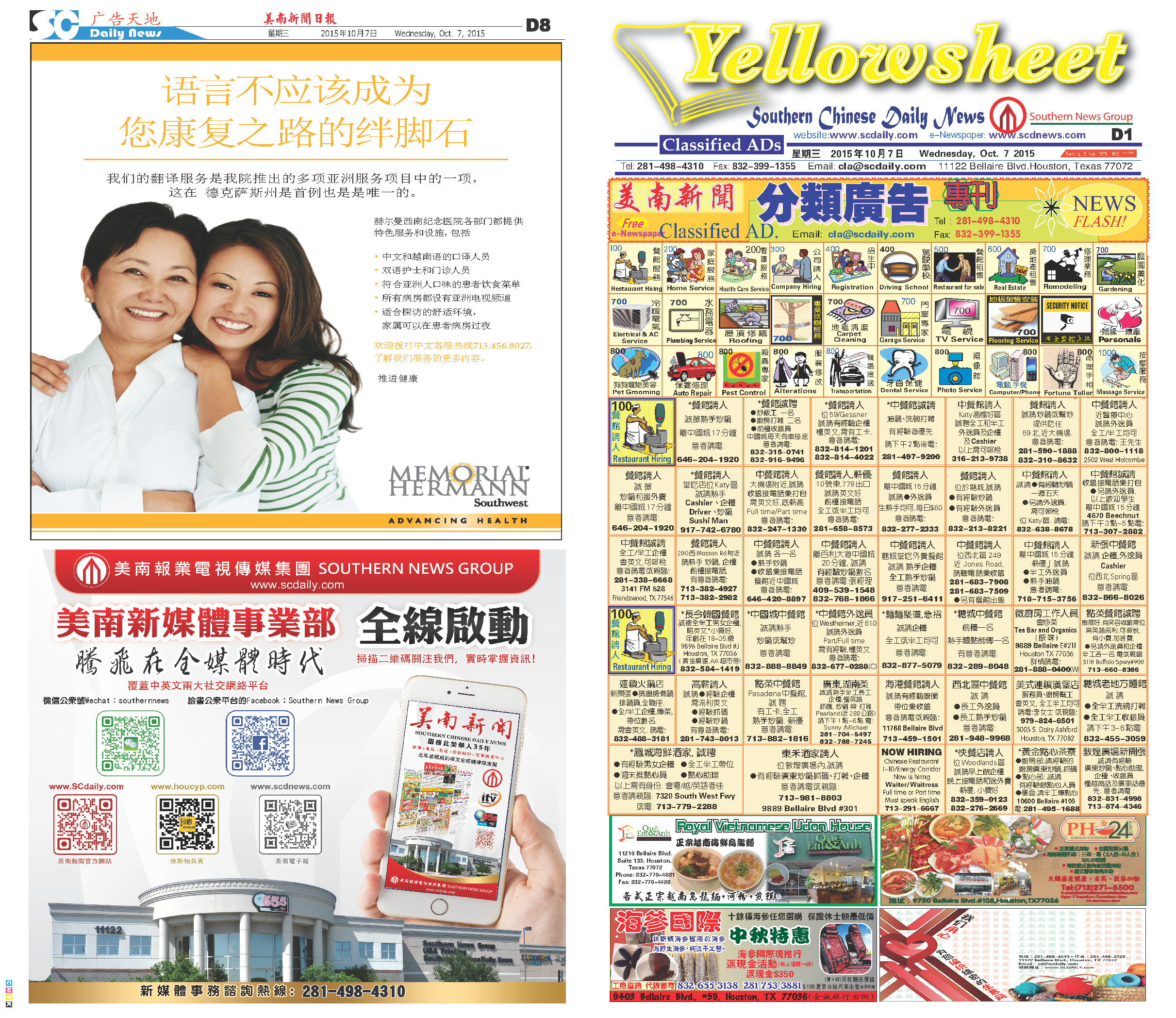151017 Epaper

 A Section B SectionC Section D Section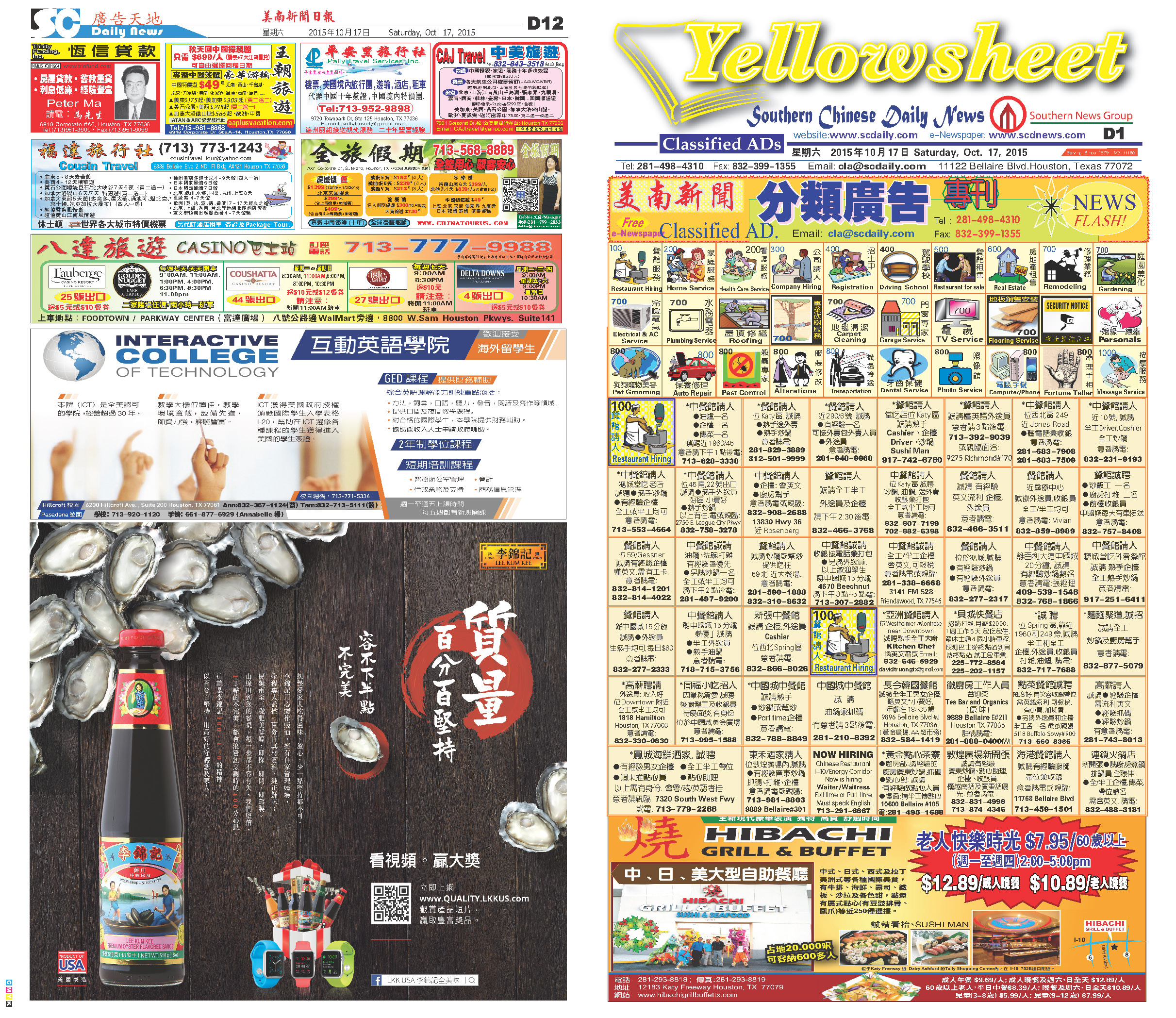151016 Epaper

 A Section B SectionC Section D Section151015 Epaper

 A Section B SectionC Section D Section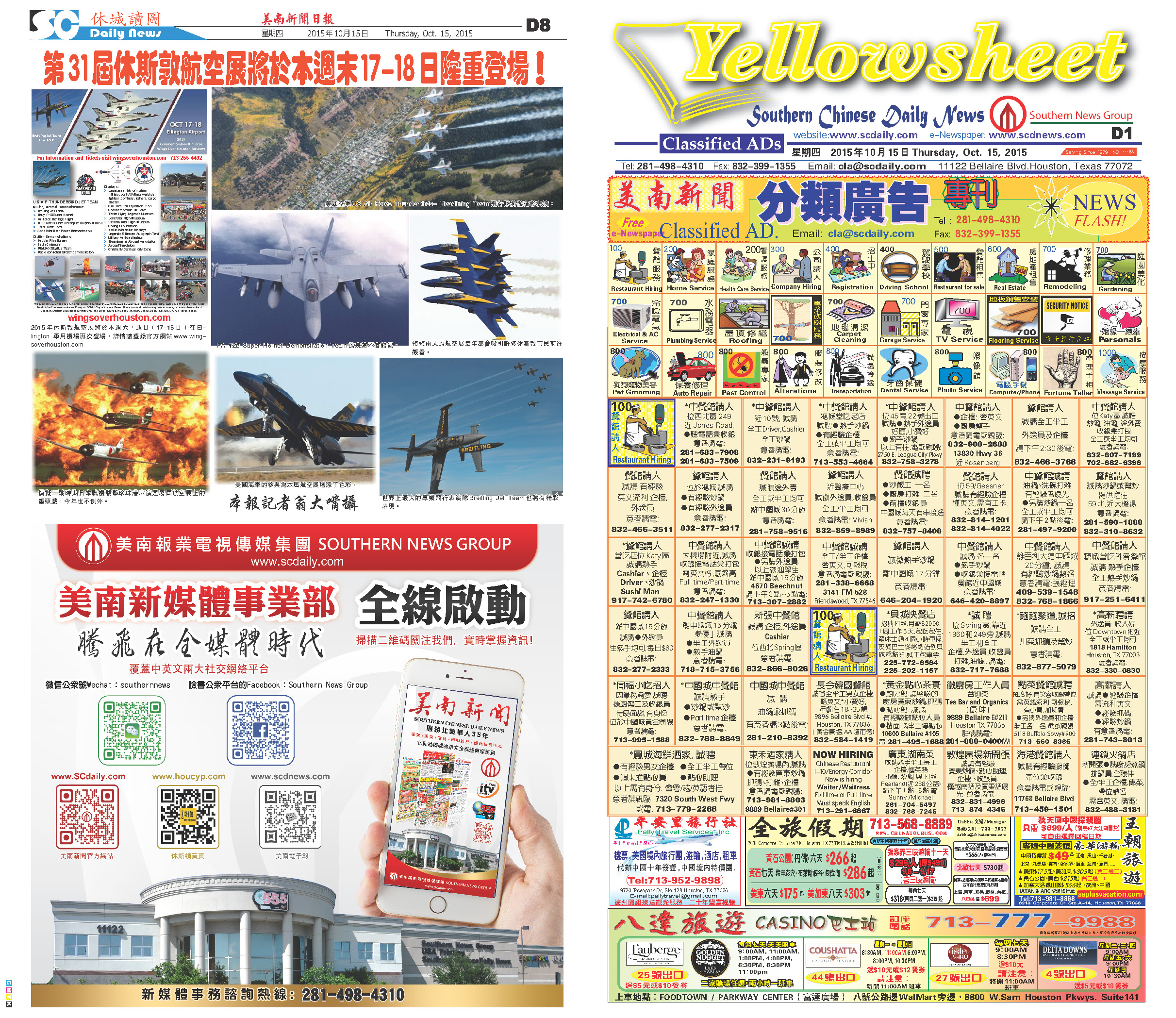151014 Epaper

 A Section B SectionC Section D Section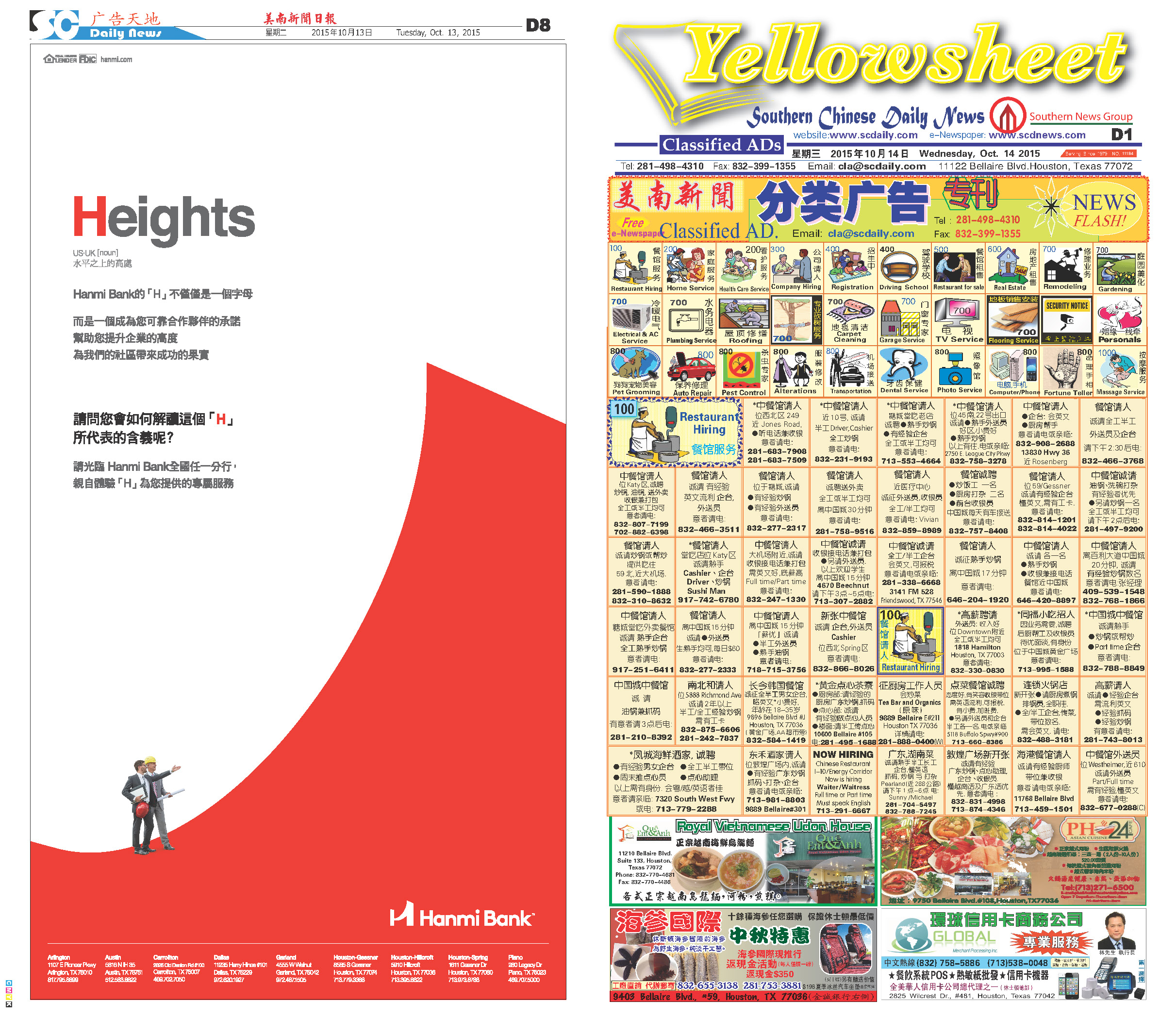151013 Epaper

 A Section B SectionC Section D Section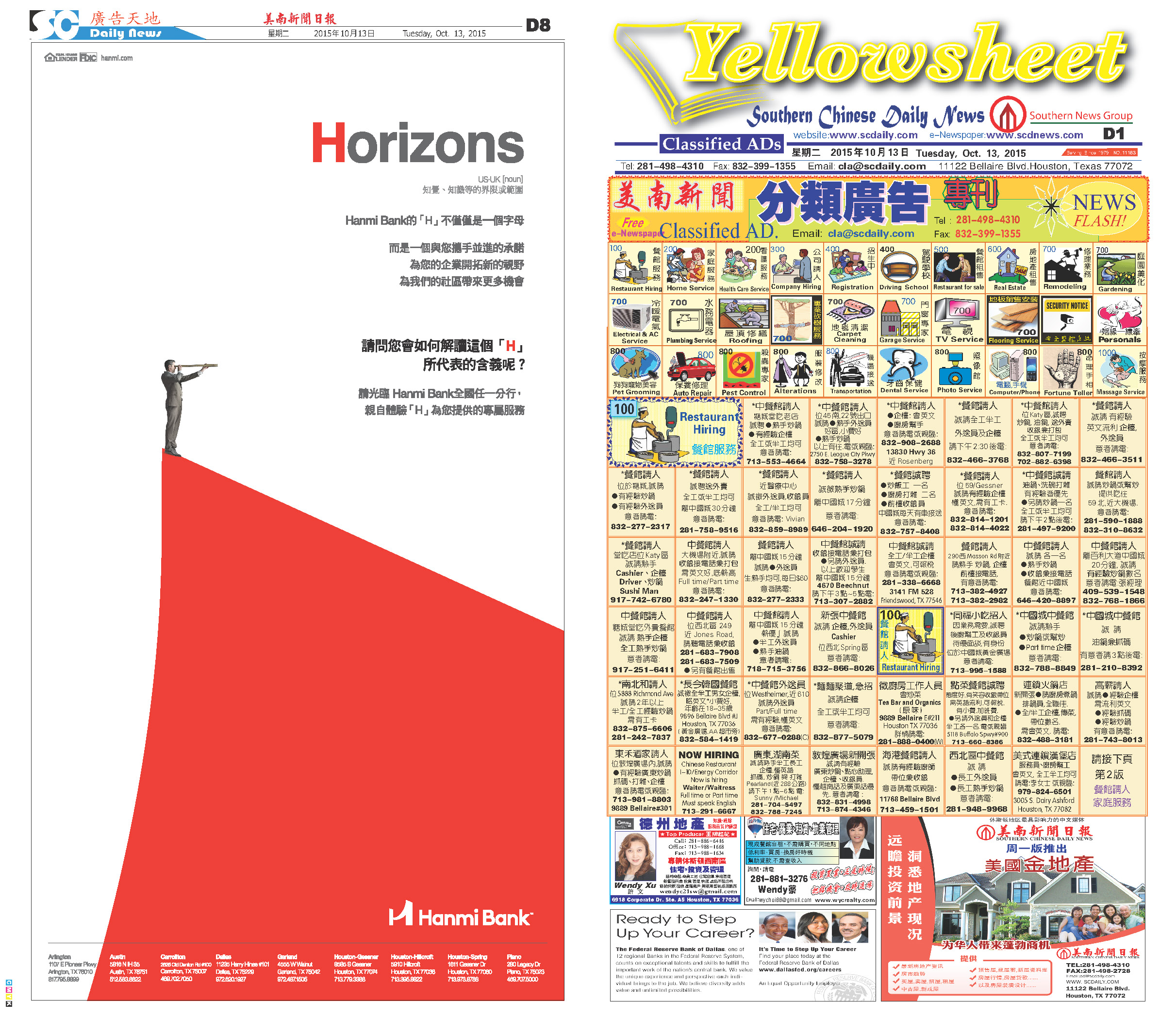151012 Epaper

 A Section B SectionC Section D Section151011 Epaper

 A Section B SectionC Section D Section151010 Epaper

 A Section B SectionC Section D Section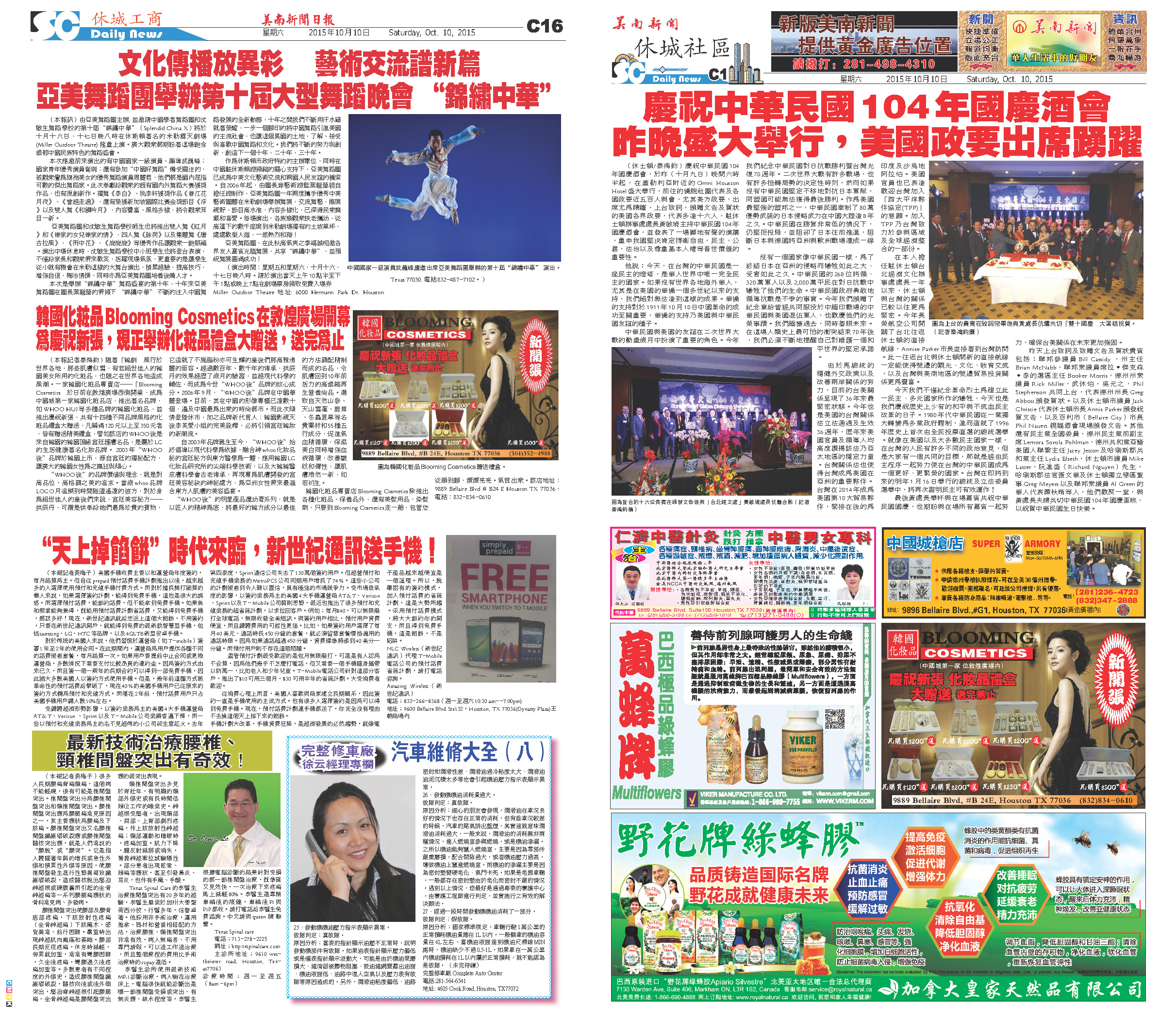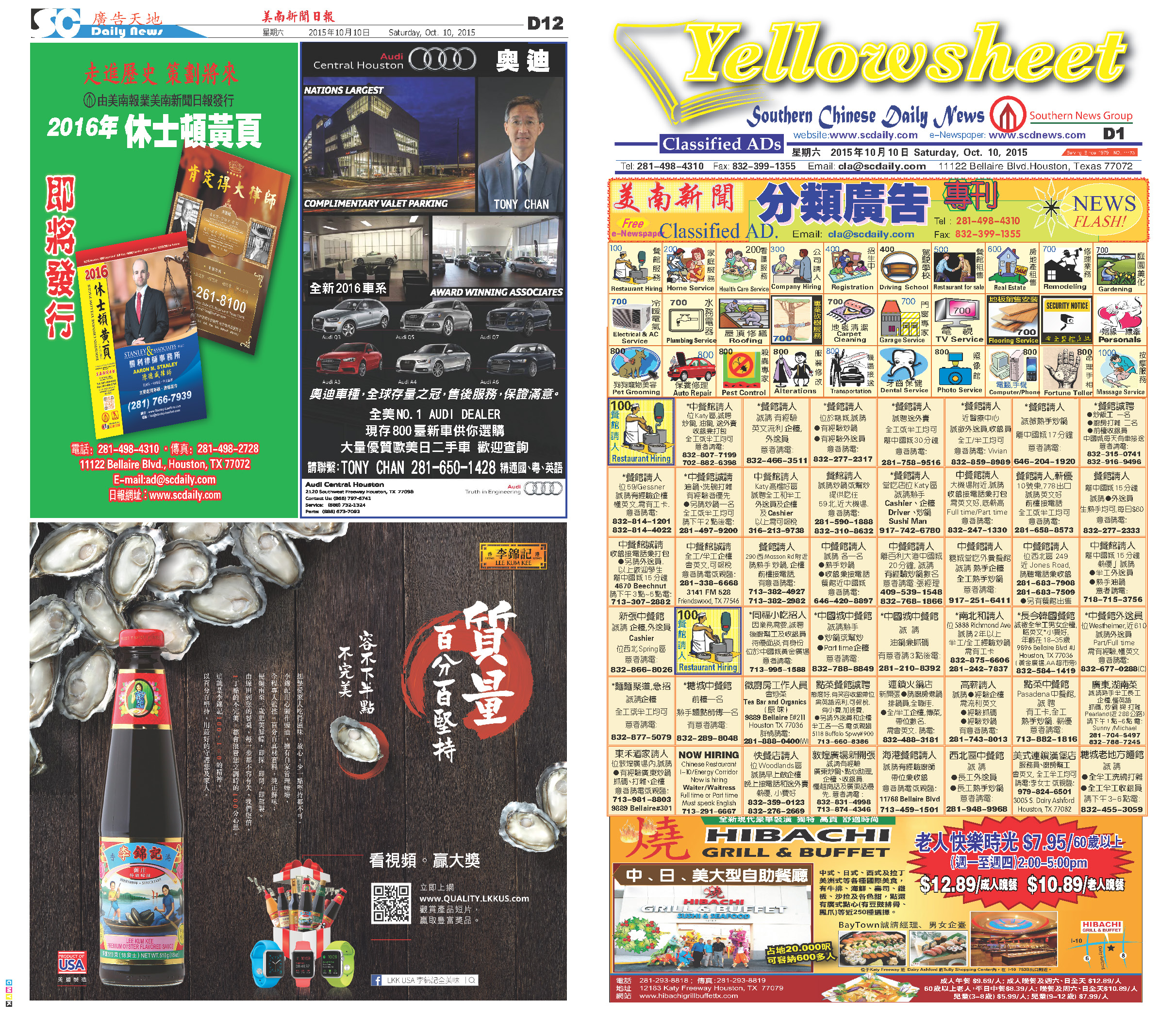151008 Epaper

 A Section B SectionC Section D Section151009 Epaper

 A Section B SectionC Section D Section151007 Epaper

 A Section B Section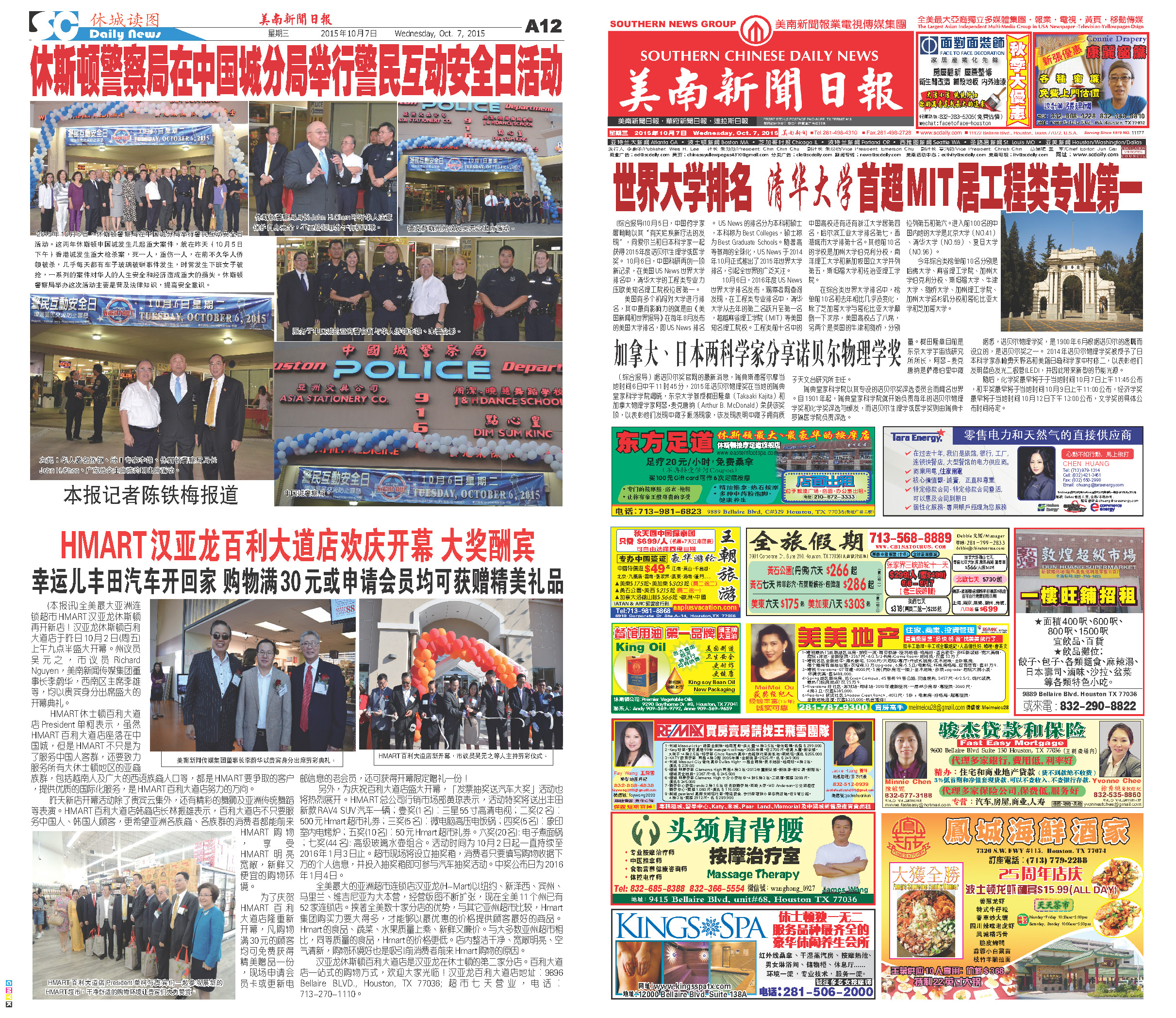C Section D Section